# Vector Direction and Bearing

 Bearing Bearing is used to give direction in aviation. It is defined as a positive angle from 0 to 360 measured clockwise with respect to the north.(see in figure 1 examples below)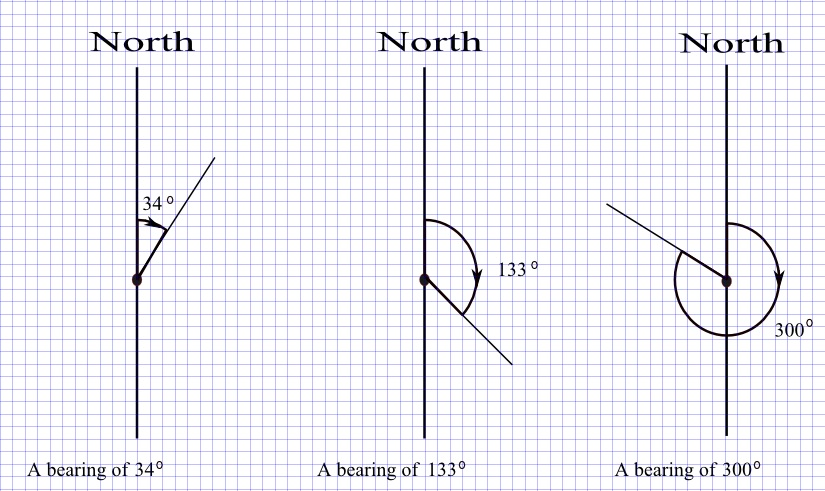Fig1. - Examples of Bearing Directions. Magnetic Compass Magnetic compass was used by sailors to give directions.Using the four cardinal points of a compass, a direction is given as an angle of rotation from one one cardinal to another. Examples are given in figure 2 below. Example on the left, the direction "W 30� N" is the angle of rotation of 30�, from West to North. Example on the right, the direction "N 60� E" is the angle of rotation of 60� from North to East.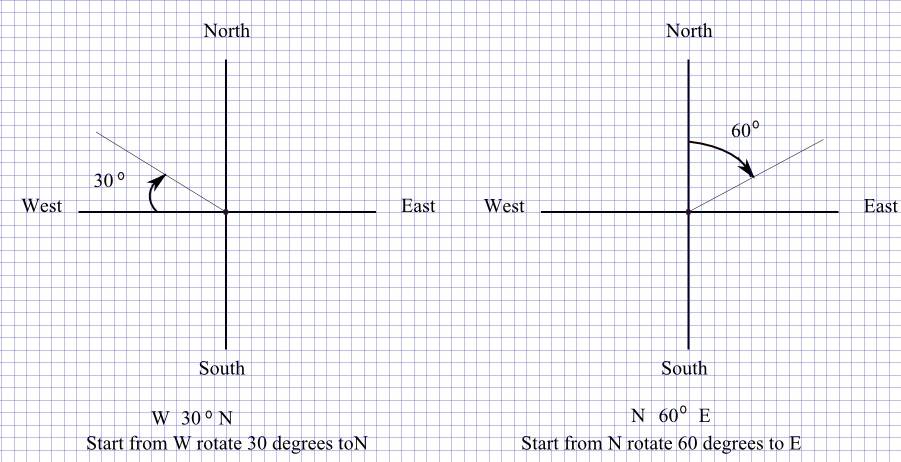Fig2. - Examples of Directions Given Using Compass. Applications of Bearing Question 1 Find the components of vector OA where O is the origin of the system of rectangular axes and A is a point 20 units away from the origin at a bearing of 30�. Solution to Question 1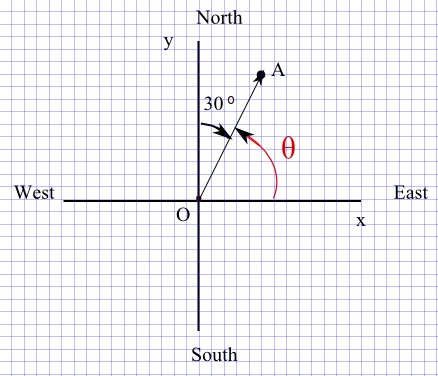Fig3. - Diagram for question 1. We first make a diagram including point A at the given bearing and determine the size of the angle between the positive x-axis and vector OA. (see figure 1 on the right) θ = 90� - 30� = 60� We also know that the length of vector OA is 10 units. Hence the x and y components of vector OA are respectively given by Ax = 10 cos(60�) = 5 Ay = 10 sin(60�) = 5 √3 Question 2 A ship traveled a distance of 50 km from port O at a bearing of 120�, then another 100 km to port B at a bearing of 200 �. What is the distance between ports O and B? Solution to Question 2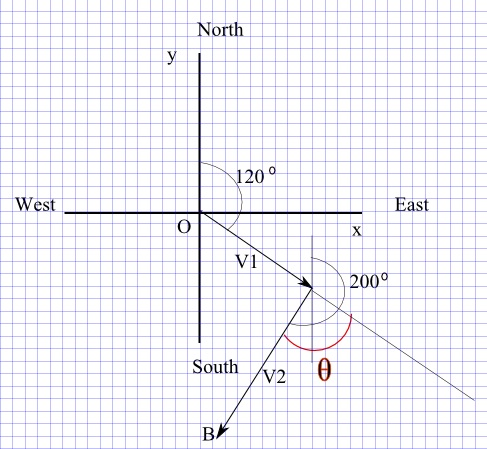Fig4. - Diagram for question 2. A diagram including the path followed by the ship is shown on the right. (see figure). V1 is the vector describing the direction and distance of the first part of the journey (50 km) and V2 is the vector describing the direction and distance of the second part of the journey (100 km). To find the distance from O to B, we may use vector OB as follows OB = V1 + V2 OB�OB = (V1 + V2)�(V1 + V2) Note that: OB�OB = OB 2 Hence: OB 2 = V1 2 + V2 2 + 2V1�V2 V1�V2 = V1 V2 cos (θ), where θ is the angle between V1 and V2 as shown in figure 2 and is equal to θ = 200� - 120� = 80� Hence OB 2 = 50 2 + 100 2 + 2 (50)(100)cos(80�) OB = √( 50 2 + 100 2 + 2 (50)(100)cos(80�) ) ≈ 119.31 km Question 3 Point A is 20 units away from the origin in the direction "N 30� W" and point B is 30 units away from the origin in the direction "S 58� W". Find the components of vector AB. Solution to Question 3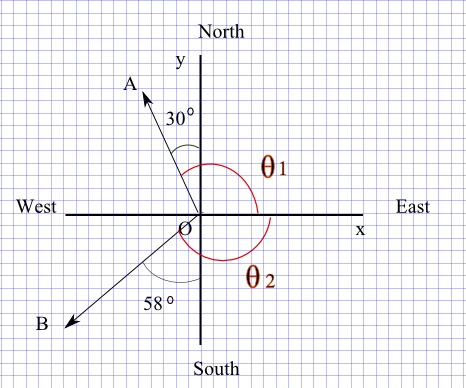Fig5. - Diagram for question 3. Vector AB may be expressed as AB = AO + OB Let us find the components of vectors OA and OB. OA = (20 cos(θ1) , 20 sin(θ1)) = ( 20 cos(120�) , 20 sin(120�) ) OB = ( 30 cos(θ2) , 30 sin(θ2) ) = 30 cos(-148�) , 30 sin(-148�) ) Note that AO = -OA = ( -20 cos(120�) , -20 sin(120�) ) We now calculate the components of vector AB as follows AB = AO + OB = ( -20 cos(120�) , -20 sin(120�) ) + (30 cos(-148�) , 30 sin(-148�) ) = ( -20 cos(120�) + 30 cos(-148�) , -20 sin(120�) + 30 sin(-148�) ) ≈ (-15.44 , -33.21) Question 4 Find the components of a unit vector U whose direction is along the bearing of 30�. Solution to Question 4 By definition, a unit vector has a magnitude equal to 1. The direction of the unit vector U is along the bearing of 30�. Therefor the angle between vector U and the positive x-axis is 60�. Hence the components of vector U are given by Ux = (1) cos(60�) = 1/2 Uy = (1) sin(60�) = √ 3 / 2 Question 5 An object moves from the origin O to point A to B and finally stops at point C (see figure below). The distances covered are: OA = 10 cm, AB = 12 cm and BC = 20 cm. The directions are given by the bearings as follows: 120� from O to A , 180� from A to B and 250� from B to C .Find the distance from O to C. Solution to Question 5Fig6. - Diagram for question 5. Vector OC may be written as the sum of three vectors as follows OC = OA + AB + BC The angles between the three vectors and the positive x-axis are Vector OA : θ1 = -(120� - 90�) = -30� Vector AB : θ2 = -(180� - 90�) = -90� Vector BC : θ3 = -(250� - 90�) = -160� We now know the magnitudes and directions of each of three vectors; calculate the components of each vector OA = (10 cos(-30) , 10 sin(-30)) AB = (12 cos (-90) , 12 sin (-90)) = (0 , -12) BC = (20 cos (-160) , 20 sin (-160) ) The components of vector OC are given by the sum of the components of the 3 vectors OA, AB and BC vector OC = ( 10 cos(-30) + 0 + 20 cos (-160) , 10 sin(-30) - 12 + 20 sin (-160)) Distance OC is given by the magnitude of vector OC distance OC = √( (10 cos(-30) + 0 + 20 cos (-160))2 + (10 sin(-30) - 12 + 20 sin (-160))2) ≈ 25.46